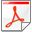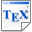[BdAFK16] C. Baier, L. de Alfaro, V. Forejt, and M. Kwiatkowska. Probabilistic Model Checking. In Handbook of Model Checking, S​pringer. To appear. 2016. [pdf] [bib] Downloads:pdf (277 KB)bib Notes: The publication will be available from Springer, the linked copy is authors' copy. Links: [Google] [Google Scholar] [CiteSeer] Abstract. The model-checking approach was originally formulated for verifying qualitative properties of systems, for example safety and liveness, and subsequently extended to also handle quantitative features, such as real-time, continuous flows, as well as stochastic phenomena, where system evolution is governed by a given probability distribution. Probabilistic model-checking aims to establish the correctness of probabilistic system models against quantitative probabilistic specifications, such as those capable of expressing, e.g., the probability of an unsafe event occurring, expected time to termination, or expected power consumption in the start-up phase. In this chapter, we present the foundations of probabilistic model-checking, focusing on finite-state Markov decision processes as models and quantitative properties expressed in probabilistic temporal logic. Markov decision processes can be thought of as a probabilistic variant of labelled transition systems in the following sense: transitions are labelled with actions, which can be chosen nondeterministically, and successor states for the chosen action are specified by means of discrete probabilistic distributions, thus specifying the probability of transiting to each successor state. To reason about expectations, we additionally annotate Markov decision processes with quantitative costs, which are incurred upon taking the selected action from a given state. Quantitative properties are expressed as formulas of the probabilistic computation tree logic (PCTL) or using linear temporal logic (LTL). We summarise the main model-checking algorithms for both PCTL and LTL, and illustrate their working through examples. The chapter ends with a brief overview of extensions to more expressive models and temporal logics, existing probabilistic model-checking tool support, and main application domains.